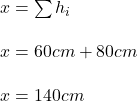Question

Two water waves meet at the same point, one having a displacement above equilibrium of 60 cm and the other having a displacement above equilibrium of 80 cm.

1.thuhuong

The resultant displacement is 140 cm

Explanation:

say first displacement d₁ is 60 cm

and second displacement d₂ is 80 cm

Resultant displacement = d₁ + d₂

= 60 + 80

= 140 cm

2.